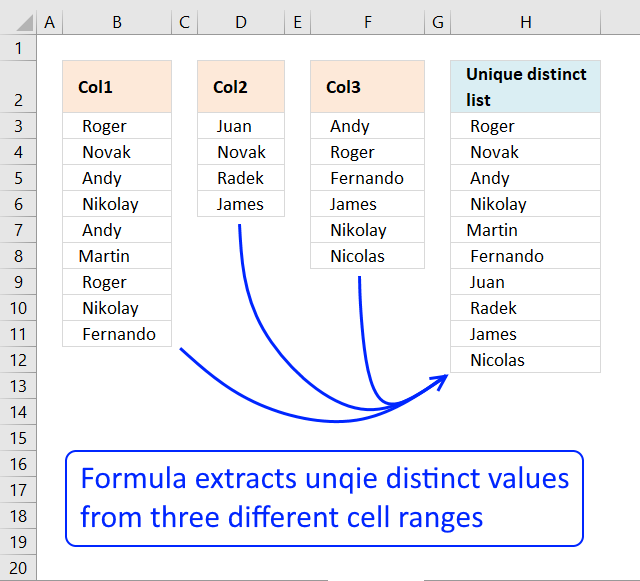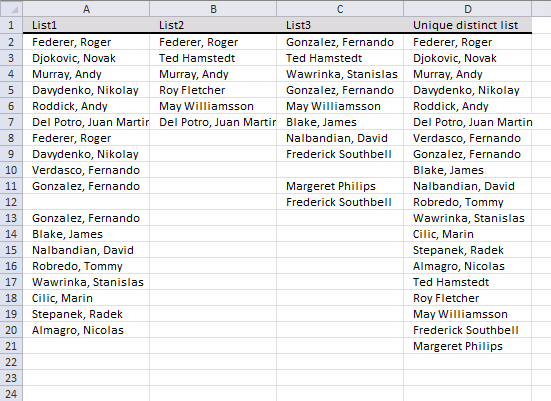Author: Oscar Cronquist Article last updated on November 19, 2018Question: How do I extract a unique distinct list from three ranges or lists? The ranges are not necessarily adjacent or the exact same length.

### Extract a unique distinct list from three columns

This formula consists of three similar parts, one returns values from Col1, the second one from col2 and the third from Col3.

IFERROR(IFERROR(formula1, formula2), formula3)

Formula in H3:

=IFERROR(IFERROR(LOOKUP(2, 1/(COUNTIF(\$H\$2:H2,\$B\$3:\$B\$11)=0), \$B\$3:\$B\$11), LOOKUP(2, 1/(COUNTIF(\$H\$2:H2, \$D\$3:\$D\$6)=0), \$D\$3:\$D\$6)),LOOKUP(2, 1/(COUNTIF(\$H\$2:H2, \$F\$3:\$F\$8)=0), \$F\$3:\$F\$8))

### Explaining formula in cell H3

#### Step 1 - Prevent duplicate values

The COUNTIF function counts values based on a condition, in this case, I am counting values in cells above. This makes sure that duplicates are ignored.

COUNTIF(\$H\$2:H2,\$B\$3:\$B\$11)=0

becomes

COUNTIF("Unique distinct list",{" Roger ";" Novak ";" Andy ";" Nikolay ";" Andy ";"Martin ";" Roger ";" Nikolay ";" Fernando "})=0

becomes

{0;0;0;0;0;0;0;0;0}=0

and returns

{TRUE;TRUE; TRUE;TRUE; TRUE;TRUE; TRUE;TRUE; TRUE}

#### Step 2 - Divide 1 with array

The LOOKUP function ignores error and if we divide 1 with 0 an error occurs. 1/0 = #DIV/0!

1/(COUNTIF(\$F\$2:F2,\$B\$3:\$B\$11)=0)

becomes

1/{TRUE;TRUE; TRUE;TRUE; TRUE;TRUE; TRUE;TRUE; TRUE}

and returns

{1;1;1;1;1;1;1;1;1}

#### Step 3 - Return value based on array

LOOKUP(2, 1/(COUNTIF(\$F\$2:F2,\$B\$3:\$B\$11)=0), \$B\$3:\$B\$11)

becomes

LOOKUP(2, {1;1;1;1;1;1;1;1;1}, \$B\$3:\$B\$21)

becomes

LOOKUP(2, {1;1;1;1;1;1;1;1;1}, {" Roger ";" Novak ";" Andy ";" Nikolay ";" Andy ";"Martin ";" Roger ";" Nikolay ";" Fernando "})

and returns " Fernando " in cell F3.

#### Step 4 - Return values from Col2

When values run out from Col1 formula1 returns errors, the IFERROR function then moves to formula2.

IFERROR(IFERROR(formula1, formula2), formula3)

formula2 is just like formula1 except that it returns values from Col2 etc.

### Extract a unique distinct list from three columns with possible blanksArray formula in D2:

=IFERROR(IFERROR(IFERROR(INDEX(\$A\$2:\$A\$20, MATCH(0, COUNTIF(\$D\$1:D1, \$A\$2:\$A\$20)+(\$A\$2:\$A\$20=""), 0)), INDEX(\$B\$2:\$B\$7, MATCH(0, COUNTIF(\$D\$1:D1, \$B\$2:\$B\$7)+(\$B\$2:\$B\$7=""), 0))), INDEX(\$C\$2:\$C\$12, MATCH(0, COUNTIF(\$D\$1:D1, \$C\$2:\$C\$12)+(\$C\$2:\$C\$12=""), 0))), "")

### How to enter an array formula

1. Select cell D2
2. Press with left mouse button on in formula bar
3. Paste array formula to formula bar
4. Press and hold CTRL + SHIFT
5. Press ENTER

### Get Excel *.xlsx file

how-to-extract-a-unique-list-from-three-columns-in-excelv3.xlsx